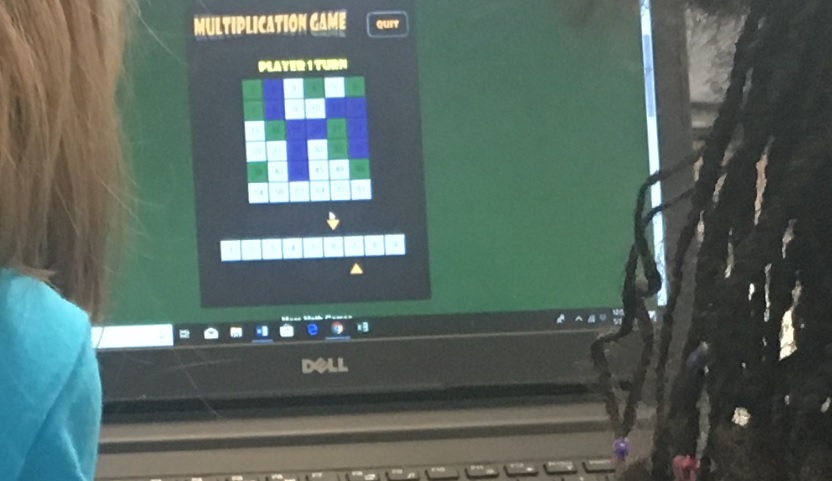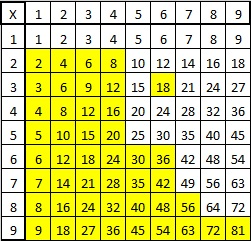# 1381 Hooda Math’s Multiplication Game

The school year is almost over, and class periods were only twenty-five minutes long today. I went online looking for math games that would benefit my students and I found a winner with Hooda Math’s Multiplication Game.If you count the multiplication facts in a 9×9 multiplication table, you will see 99 facts, but many of the products are duplicated in the table. Every yellow square below is also in white elsewhere in the table:There are actually only 36 unique products in the multiplication table above. Hooda Math has cleverly arranged those 36 products in a 6×6 grid that becomes the game board. In this two-person game, students take turns moving one of two arrows to a number from 1 to 9 at the bottom of the screen and claiming the square that contains the product of the numbers. The catch is that players must keep one of the numbers chosen by the previous player and cannot claim a product that has already been claimed by either player. (Player 1 cannot score on his first turn.) One student is green and the other is purple and the first to claim four squares in a row is the winner. The rules on the website are VERY short and simple.

Students played this game today. I played it as well. Sometimes I won, and sometimes I lost, but the losses are more interesting than the wins:

In one game, my opponent took the square that I needed to get four in a row vertically for the win. All she was trying to do was block me from winning, however, when she took that square, the game declared her the winner. We were puzzled why she was the winner until she figured out that making that move gave her four in a row diagonally. That’s when we found out players can win by getting four in a row diagonally as well as vertically or horizontally.

In another game, I had two possible moves that would have made me be the winner. I just needed my opponent to choose a 1, 6, or 8 as their other factor, and I would win with 1 × 1 = 1 or 6 × 8 = 48. Unfortunately, he knew to beware of the numbers that would make me win. One of the arrows was pointing to 5, and he made the other arrow point to 5. By now there were no other products left on the board that were divisible by 5, so I couldn’t win because I couldn’t move either of the arrows.

That’s how I didn’t win the game either of those times, but I had a lot of fun anyway, and you will, too!

This is my 1381st post. Here’s some information about that number:

• 1381 is a prime number.
• Prime factorization: 1381 is prime.
• 1381 has no exponents greater than 1 in its prime factorization, so √1381 cannot be simplified.
• The exponent in the prime factorization is 1. Adding one to that exponent we get (1 + 1) = 2. Therefore 1381 has exactly 2 factors.
• The factors of 1381 are outlined with their factor pair partners in the graphic below.How do we know that 1381 is a prime number? If 1381 were not a prime number, then it would be divisible by at least one prime number less than or equal to √1381. Since 1381 cannot be divided evenly by 2, 3, 5, 7, 11, 13, 17, 19, 23, 29, 31, or 37, we know that 1381 is a prime number.

1381 is the 24th Centered Pentagonal Number because
5(23)(24)/2 + 1 = 1381. In formula means that it is one more than five times the 23rd triangular number, as I’ve illustrated below:1381 is the sum of two squares:
34² +15² = 1381

1381 is the hypotenuse of a Pythagorean triple:
931-1020-1381 calculated from 34² -15², 2(34)(15), 34² +15²

Here’s another way we know that 1381 is a prime number: Since its last two digits divided by 4 leave a remainder of 1, and 34² + 15² = 1381 with 34 and 15 having no common prime factors, 1381 will be prime unless it is divisible by a prime number Pythagorean triple hypotenuse less than or equal to √1381. Since 1381 is not divisible by 5, 13, 17,  29, or 37, we know that 1381 is a prime number.

This site uses Akismet to reduce spam. Learn how your comment data is processed.